## Logistic Equation r=4

With, the Logistic Equation becomes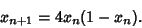(1)

Now let(2)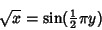(3)(4)(5)

Manipulating (2) gives(6)

so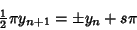(7)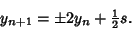(8)

But. Taking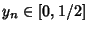, thenand(9)

For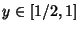,and(10)

Combining(11)

which can be written(12)

the Tent Map with, so the Natural Invariant inis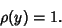(13)

Transforming back togives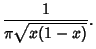(14)

This can also be derived from(15)

where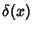is the Delta Function.